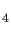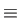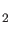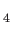### Keyword CONSTRAINTS

This keyword specifies molecular dynamics constraints.
Options: None
Description:
Molecular dynamics constraints are introduced force neutral along interatomic connection lines with the Rattle  and Shake  algorithms. They can be defined in terms of internal coordinates, i.e. bond lengths, bond angles and dihedral angles, in the keyword body of the CONSTRAINTS keyword. In the following example the bond length between atoms 1 and 3 (C-H2), the bond angle between atoms 1, 2 and 7 (C-H1-O) and the dihedral angle between atoms 1, 3, 7 and 8 (C-H2-O-H) are constrained during a BOMD simulation of CHand HO.

 DYNAMICS STEP=0.5 MAX=200 INT=1
CONSTRAINTS
1 3
1 2 7
1 3 7 8
PRINT CONSTRAINTS
BATH NOSE NHC=3  T=300
VELOCITIES RANDOM LP=0
LPCONSERVE FORCES
VISUALIZE MOLDEN MD
#
GEOMETRY CARTESIAN  ANGSTROMS
C     0.000000     0.000000     0.000000
H1    0.000000     0.000000     1.092841
H2    1.033412     0.000000    -0.357505
H3   -0.517308     0.895483    -0.357014
H4   -0.518495    -0.891704    -0.361515
H     0.521017     0.588124    -2.579748
O     0.928735     0.380831    -3.434937
H     0.920449    -0.588239    -3.473172


As this example shows, each constraint is defined by a set of integer numbers in a line of the CONSTRAINTS keyword body. Alternatively, it is possible to constrain an entire set of atoms as shown in the following example.

 DYNAMICS STEP=0.5 MAX=200 INT=1
CONSTRAINTS
1 - 5
PRINT CONSTRAINTS
BATH NOSE NHC=3  T=300
VELOCITIES RANDOM LP=0
LPCONSERVE FORCES
VISUALIZE MOLDEN MD
#
GEOMETRY CARTESIAN  ANGSTROMS
C     0.000000     0.000000     0.000000
H1    0.000000     0.000000     1.092841
H2    1.033412     0.000000    -0.357505
H3   -0.517308     0.895483    -0.357014
H4   -0.518495    -0.891704    -0.361515
H     0.521017     0.588124    -2.579748
O     0.928735     0.380831    -3.434937
H     0.920449    -0.588239    -3.473172


Here the first five atoms (the CHmolecule) are constrained during the BOMD simulation. Note that dummy atoms must be taken into account for the atom numbering in constraints as the following example shows.

 DYNAMICS STEP=1.0 MAX=100 R=6.0 INT=1
PRINT CONSTRAINTS
BASIS
C (STO-3G)
H (DZVP)
N (STO-3G)
AUXIS
C (A2)
H (A2)
N (GEN-A2)
MULTIPLICITY 1
VXCTYPE PBE
TRAJECTORY RESTART
BATH NOSE T=500 NHC=3 FREQ=200
VELOCITIES RANDOM LP=0 T=500
LPCONSERVE ON
GUESS RESTART
VISUALIZATION MOLDEN MD
#
# Z-Matrix coordinates of MD step 100
#
GEOMETRY Z-MATRIX BOHR
C                                      6    12.011000
H   1  RCH                             1     1.007940
X   1  RCX   2  A1              RAD    0     0.000000
N   1  RCN   3  A2    2  DX1    RAD    7    14.006740
#
VARIABLES
RCH         2.06518100
RCX         1.89096462
RCN         2.27049634
A1           89.538122
A2           89.876160
DX1         163.715357
#
CONSTRAINTS
1      2
1      4


In this input the C-H and CN bond lengths in HCN are fixed during the BOMD. The CONSTRAINTS keyword can also be used for MM MD simulations. The following example shows an MM MD input for (HO)in which the first water molecule is kept frozen.

 LPCONSERVE
CONSTRAINTS
1 - 3
VISUALIZATION MD
PRINT CONSTRAINTS
DISPERSION S6=1.0
BATH NOSE NHC=3 T=300
VELOCITIES RANDOM LP=0
FORCEFIELD FF=OPLS-AA
DYNAMICS STEP=0.5 MAX=1000 INT=1
#
GEOMETRY MIXED ANGSTROM
O1   1.187632     0.332759    -1.195914    -76 2 3
H2   0.217713     0.505507    -1.369657    -77 1
H3   1.503324    -0.129952    -1.999029    -77 1
O4  -1.628323     0.600651    -1.413703    -76
H5  -1.885969     0.153697    -0.557057    -77
H6  -2.021673     1.495719    -1.360149    -77
O7   0.867185    -1.043515     1.275905    -76 8 9
H8   1.124357    -0.596134     0.419304    -77 7
H9   1.262389    -1.937796     1.222724    -77 7
O10 O7  R1  O4  A1  O1  D1  -76 11 12
H11 O10 R2  O4  A2  O1  D2  -77 10
H12 O10 R3  H11 A3  O7  D3  -77 10
#
Variables
R1  3.0
R2  1.0
R3  1.0
A1 90.0
A2 10.0
A3 99.0
D1  0.0
D2 45.0
D3 90.0


The extension of the CONSTRAINTS keyword to QM/MM molecular dynamics is straightforward. It can be applied to QM and MM atoms simultaneously.